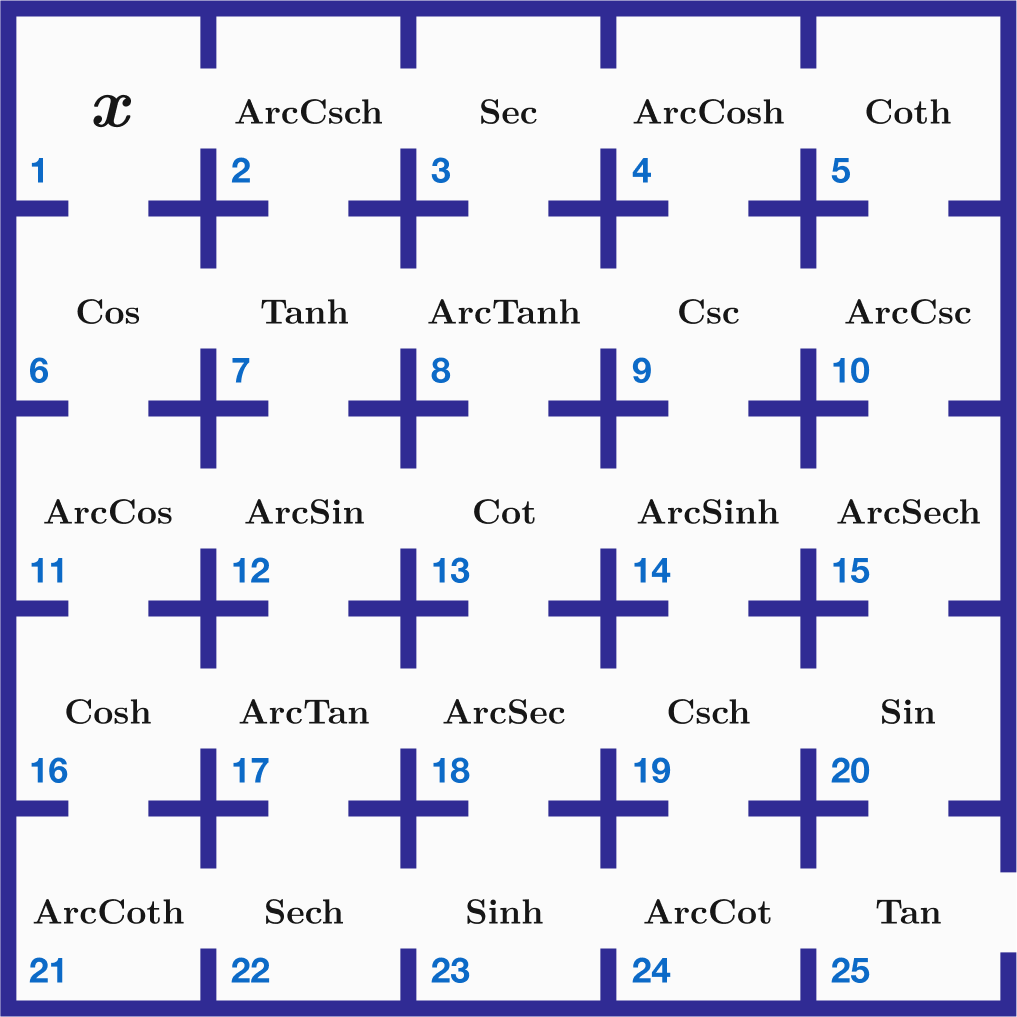# Escape From Trigonometric Maze

Calculus Level 3

You are in room $1$ in the upper left corner of a maze of rooms. The goal is to escape this maze and exit through room $25$ at the bottom right, but you can only move either right or down, passing through a total of $9$ rooms.

You need to deliberately find a path to get out exactly the same way you were when you started in room $1,$ because each room alters you, sometimes even making you imaginary.

What path will let you do that?Enter your answer as the total of the room numbers you must pass through in order to do that, a sum of $9$ numbers starting with $1$ and ending with $25$.

For example, if you go through rooms $1,2,3,4,5,10,15,20,25$, the total is $85$. However, you will experience the succession of functions
$\text{Tan}(\text{Sin}(\text{ArcSech}(\text{ArcCsc}(\text{Coth}(\text{ArcCosh}(\text{Sec}(\text{ArcCsch}(x)))))))))$ so that if you started with for example a real value of $0.5,$ you would end up being a totally imaginary number $0.714i$ when you leave the maze, which does not fulfill the requirement.

Note:

• $\large x$ cannot start with $i, -i$, or $0$ for this problem, but can be any other value, real or complex.
• See Gudermannian Function.
×